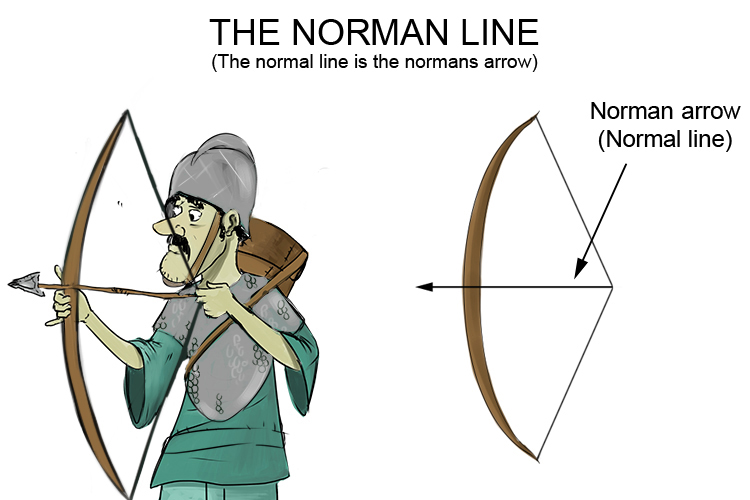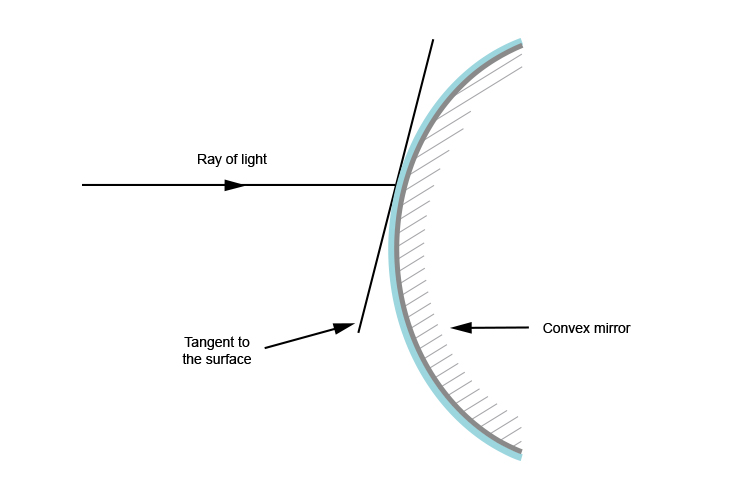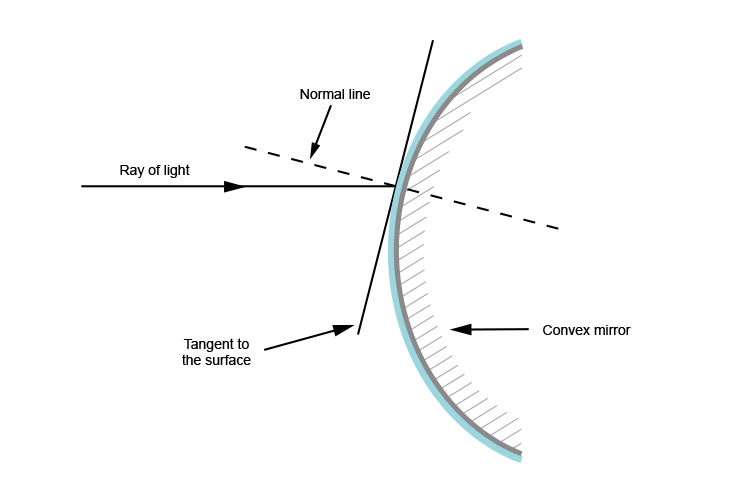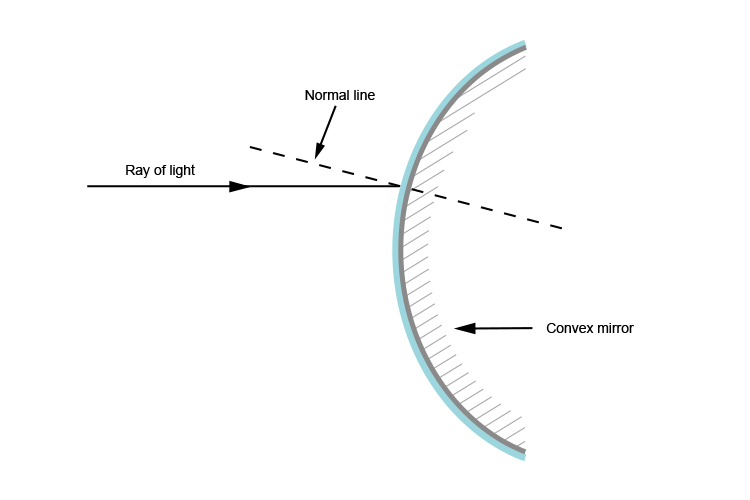# Normal line - Convex mirrors

To further understand convex mirrors, you will need to understand 'normal' line in relation to convex mirrors.

There are several definitions of the normal line of a convex mirror.

Normal line is the line perpendicular (at 90°) to the tangent line

or

The normal line is an imaginary line at 90° to where the ray touches the surface.

At Mammoth Memory, we believe it's best to think of a bow held by a Norman warrier as the curve and the Norman's arrow being the normal line.So, for a convex mirror at the point where the ray of light touches the surface, draw a tangent.Then draw a perpendicular line (at 90°) to the tangent.Then you can remove the tangent line.This is the normal line and is perpendicular to the convex mirror's surface at the point of contact.